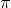## a round mirror has a circumference of 87.92 inches. calculate the area of the mirror. use 3.14 for pi. round answers to the nearest hundreth

Question

a round mirror has a circumference of 87.92 inches. calculate the area of the mirror. use 3.14 for pi. round answers to the nearest hundreth if necessary

in progress 0
6 months 2021-08-19T19:17:20+00:00 1 Answers 0 views 0

## Answers ( )

615.44 in²

Step-by-step explanation:

Use the circumference formula, C = 2r

Plug in the circumference and pi to solve for the radius, r:

C = 2r

87.92 = 2(3.14)r

87.92 = 6.28r

14 = r

Then, use the area formula, A =A = 3.14(14)²

A = 615.44 in²

So, the area of the mirror is 615.44 in²